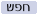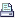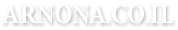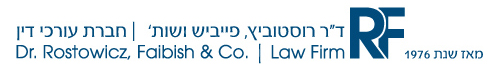ד"ר רוסטוביץ, פייביש - חברת עורכי דיןאודות ARNONAאודות העורך ד"ר הנריק רוסטוביץ

 ארכיון מגזין ארנונה 1999 - 2003השערעל סדר היוםחברה קהילה ומשפטקישוריםדבר העורךעקיצותפסקי-דיןזה קרהאז אמרו...נעשה ונשמעזה מצחיקסטיקריםאנציקלופדיה ארנונההפחתת חיובי ארנונה והיטלי פיתוחפקודת המסים (גביה)הרצאות בארנונהספרים ומאמרים
0 תגובות לכתבות מאז : 29/10/2023גרסת הדפסה

mathematics

//

From a strictly mathematical viewpoint it goes like this:
What Makes 100%?

What does it mean to give MORE than 100%?

Ever wonder about those people who say they are giving more than 100%?

We have all been to those meetings where someone wants you to give over 100%.

What makes up 100% in life?
If:
A B C D E F G H I J K L M N O P Q R S T U V W X Y Z is represented as:
1 2 3 4 5 6 7 8 9 10 11 12 13 14 15 16 17 18 19 20 21 22 23 24 25 26.

Then:
H-A-R-D-W-O-R-K
8+1+18+4+23+15+18+11 = 98%
and
K-N-O-W-L-E-D-G-E
11+14+15+23+12+5+4+7+5 = 96%
But,
A-T-T-I-T-U-D-E
1+20+20+9+20+21+4+5 = 100%
And,
B-U-L-L-S-H-I-T
2+21+12+12+19+8+9+20 = 103%
AND, look how far ass kissing will take you.
A-S-S-K-I-S-S-I-N-G
1+19+19+11+9+19+19+9+14+7 = 118%

So, one can conclude with mathematical certainty that While
Hard work and Knowledge will get you close, and Attitude will get you there, it's the Bullshit and Ass kissing that will put you over the top.

 תודה למי שיקליק על האייקון של פייסבוק

 תגובות שלח תגובה >>זכויות יוצריםד"ר רוסטוביץ, פייביש ושות' חברת עורכי דיןפורטל משפט מיסוי ונדל"ן